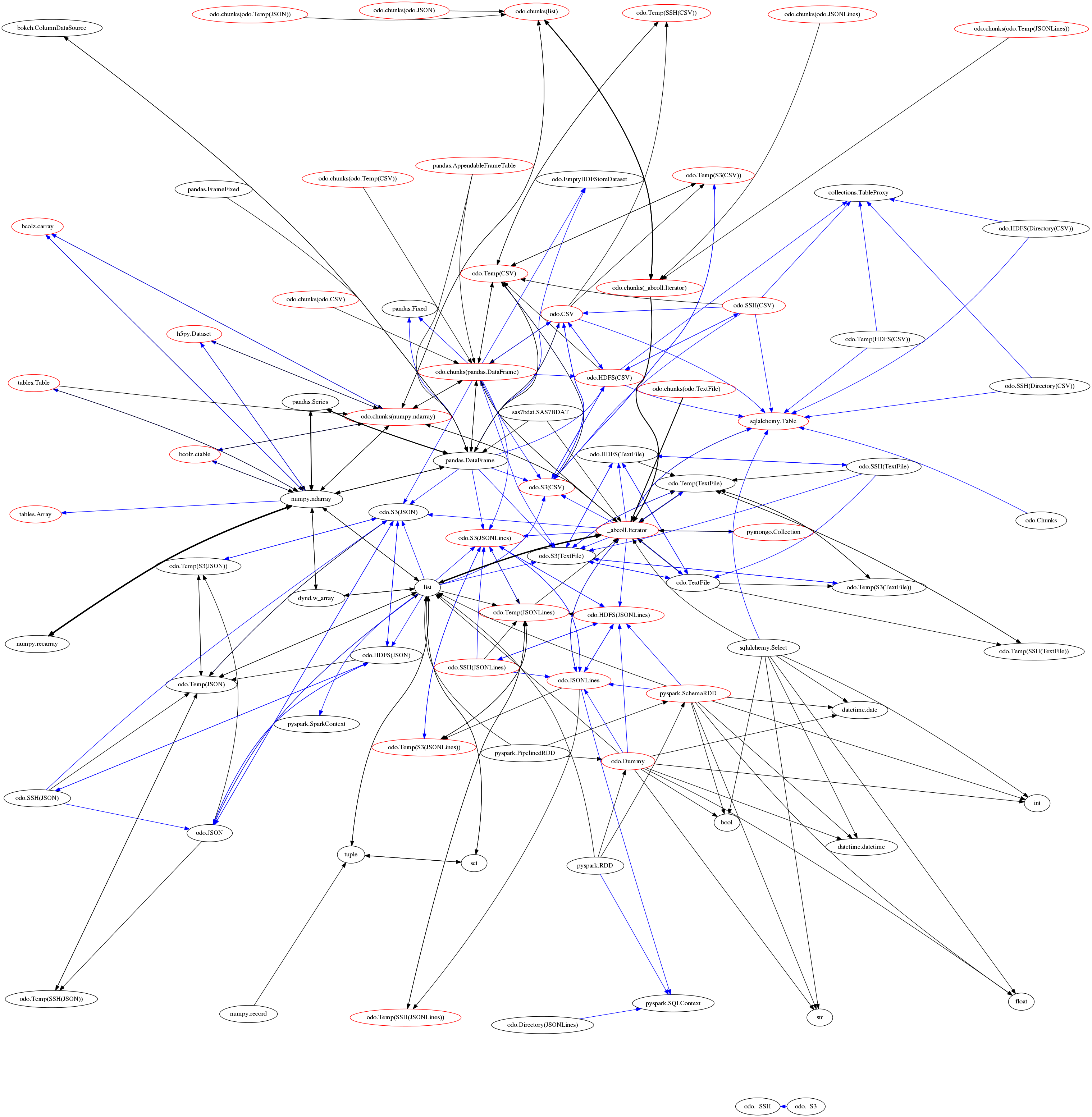{{ message }}

# blaze / odo

Data Migration for the Blaze Project

## Files

Failed to load latest commit information.
Type
Name
Commit time

# OdoData migration in Python

Documentation

## Example

Odo migrates data between different containers

```>>> from odo import odo
>>> odo((1, 2, 3), list)
[1, 2, 3]```

It operates on small, in-memory containers (as above) and large, out-of-core containers (as below)

```>>> odo('myfile.hdf5::/data', 'postgresql://user:pass@host::my-table')

Odo leverages the existing Python ecosystem. The example above uses `sqlalchemy` for SQL interation and `h5py` for HDF5 interaction.

## Method

Odo migrates data using network of small data conversion functions between type pairs. That network is below:Each node is a container type (like `pandas.DataFrame` or `sqlalchemy.Table`) and each directed edge is a function that transforms or appends one container into or onto another. We annotate these functions/edges with relative costs.

This network approach allows `odo` to select the shortest path between any two types (thank you networkx). For performance reasons these functions often leverage non-Pythonic systems like NumPy arrays or native `CSV->SQL` loading functions. Odo is not dependent on only Python iterators.

This network approach is also robust. When libraries go missing or runtime errors occur `odo` can work around these holes and find new paths.

This network approach is extensible. It is easy to write small functions and register them to the overall graph. In the following example showing how we convert from `pandas.DataFrame` to a `numpy.ndarray`.

```from odo import convert

@convert.register(np.ndarray, pd.DataFrame, cost=1.0)
def dataframe_to_numpy(df, **kwargs):
return df.to_records(index=False)```

We decorate `convert` functions with the target and source types as well as a relative cost. This decoration establishes a contract that the underlying function must fulfill, in this case with the fast `DataFrame.to_records` method. Similar functions exist for `append`, to add to existing data, and `resource` for URI resolution.

• `convert`: Transform dataset into new container
• `append`: Add dataset onto existing container
• `resource`: Given a URI find the appropriate data resource
• `odo`: Call one of the above based on inputs. E.g. `odo((1, 2, 3), list) -> convert(list, (1, 2, 3))` while `L = []; odo((1, 2, 3), L) -> append(L, (1, 2, 3))`

Finally, `odo` is also aware of which containers must reside in memory and which do not. In the graph above the red-colored nodes are robust to larger-than-memory datasets. Transformations between two out-of-core datasets operate only on the subgraph of the red nodes.

## History

Odo was factored out from the Blaze project.

Data Migration for the Blaze Project

0.5.0 Latest
Apr 25, 2016

## Packages 0

No packages published

## Languages

You can’t perform that action at this time.